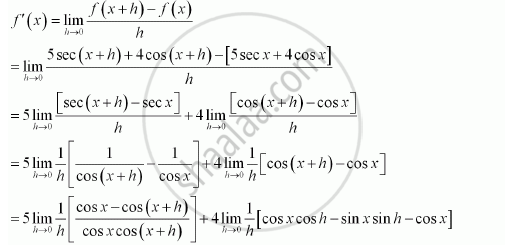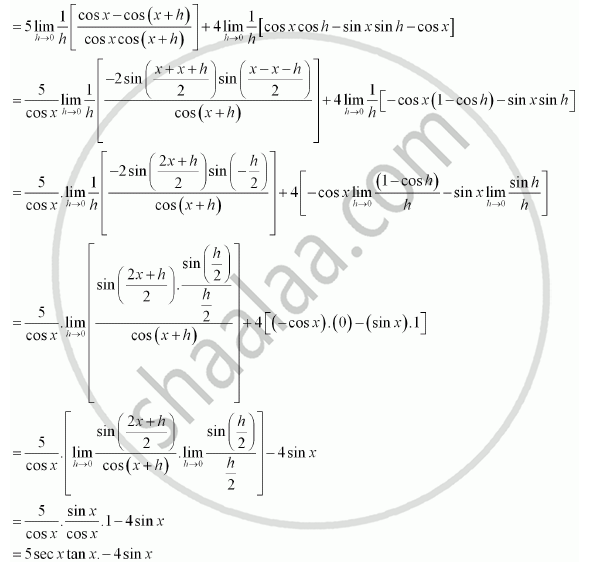CBSE (Science) Class 11CBSE
Share
Notifications

View all notifications
Books Shortlist
Your shortlist is empty

# Solution for Find the Derivative of the Following Functions: 5 Sec X + 4 Cos X - CBSE (Science) Class 11 - Mathematics

Login
Create free account

Forgot password?
ConceptDerivative Derivative of Polynomials and Trigonometric Functions

#### Question

Find the derivative of the following functions:

5 sec x + 4 cos x

#### Solution

Let f (x) = 5 sec x + 4 cos x. Accordingly, from the first principle,Is there an error in this question or solution?

#### APPEARS IN

NCERT Solution for Mathematics Textbook for Class 11 (2013 to Current)
Chapter 13: Limits and Derivatives
Q: 11.3 | Page no. 313
Solution Find the Derivative of the Following Functions: 5 Sec X + 4 Cos X Concept: Derivative - Derivative of Polynomials and Trigonometric Functions.
S